# 6:47 1 4 Search <Back Hw#3-2019.doc 15- 10. A horizontal curve is designed for a two-lane...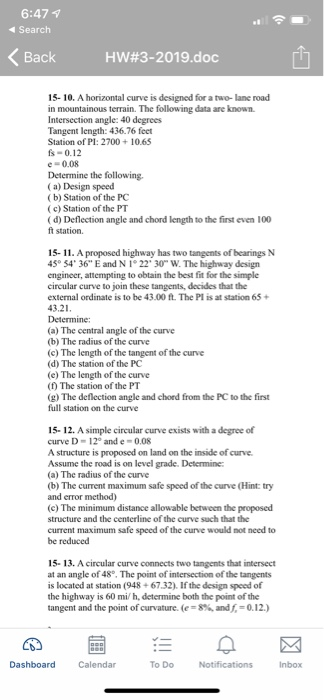6:47 1 4 Search <Back Hw#3-2019.doc 15- 10. A horizontal curve is designed for a two-lane road in mountainous terrain. The following data are known. Intersection angle: 40 degrees Tangent length: 436.76 feet Station of Pl: 2700+10.65 fs- 0.12 e- 0.08 Determine the following. (a) Design speed (b) Station of the PC (c) Station of the PT (d) Deflection angle and chord length to the first even 100 ft station. 15-11. A proposed highway has two tangents of bearings N 43° 34 36" E and N 1°22" 30 W. The highway design engineer, attempting to obtain the best fit for the simple circular curve to join these tangents, decides that the external ordinate is to be 43.00 ft. The Pl is at station 65+ 43.21 Determine: (a) The central angle of the curve (b) The radius of the curve (c) The length of the tangent of the curve (d) The station of the PC (e) The length of the curve (f) The station of the PT (g) The deflection angle and chord from the PC to the first full station on the curve curve exists with a degree of 15-12. A simple circular curve D 12° ande 0.08 A structure is proposed on land on the inside of curve Assume the road is on level grade. Determine: (a) The radius of the curve (b) The curent maximum safe speed of the curve (Hint: try and error method) (c) The minimum distance allowable between the proposed structure and the centerline of the curve such that the current maximum safe speed of the curve would not need to be reduced 15- 13. A circular curve connects two tangents that intersect at an angle of 48°. The point of intersection of the tangents is located at station (948+67.32). If the design speed of the highway is 60 mi h, determine both the point of the tangent and the point of curvature. 8%, and = 0.12.) Calendar To Do Inbox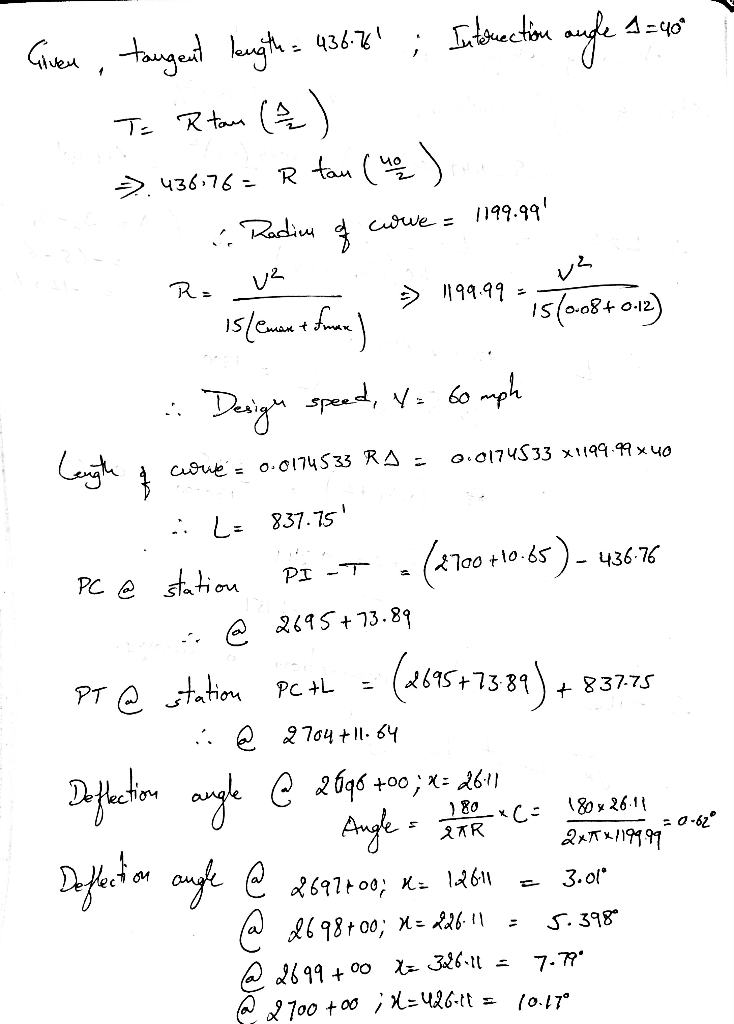#### Earn Coin

Coins can be redeemed for fabulous gifts.

Similar Homework Help Questions
• ### A horizontal curve is designed for a two-lane road in mountainous terrain. The following data is...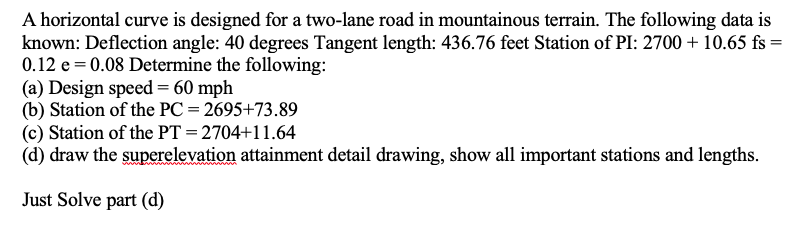A horizontal curve is designed for a two-lane road in mountainous terrain. The following data is known: Deflection angle: 40 degrees Tangent length: 436.76 feet Station of PI: 2700 10.65 fs = 0.12 e 0.08 Determine the following (a) Design speed= 60 mph (b) Station of the PC 2695+73.89 (c) Station of the PT = 2704+11.64 (d) draw the superelevation attainment detail drawing, show all important stations and lengths. wwwwnO Just Solve part (d) A horizontal curve is designed for...

• ### Problem 1 (15vpts) A proposed highway has two tangents of bearings N 75° 54' 36" E...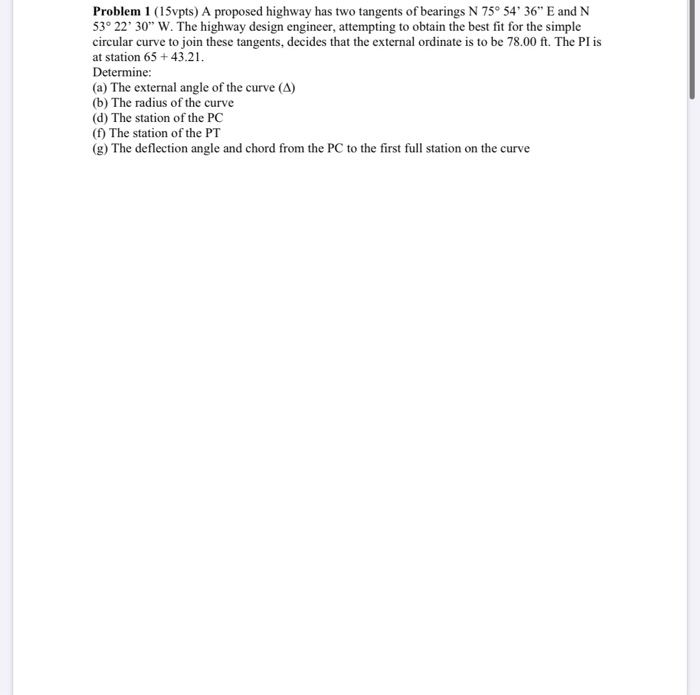Problem 1 (15vpts) A proposed highway has two tangents of bearings N 75° 54' 36" E and N 53° 22' 30" W. The highway design engineer, attempting to obtain the best fit for the simple circular curve to join these tangents, decides that the external ordinate is to be 78.00 ft. The Pl is at station 65+43.21. Determine: (a) The external angle of the curve (A) (b) The radius of the curve (d) The station of the PC (f) The...

• ### HOMEWORK CURVES Two grades g1 +1.25% and g,--2.75% intersect at station 3 +00 and the elevation...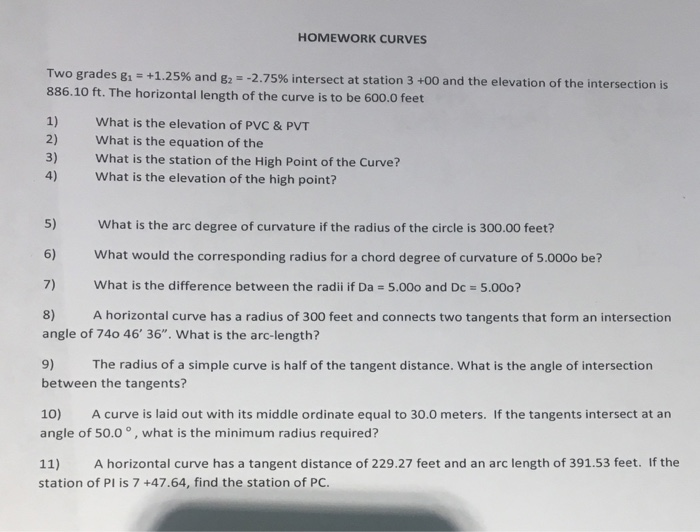HOMEWORK CURVES Two grades g1 +1.25% and g,--2.75% intersect at station 3 +00 and the elevation of the intersection is 886.10 ft. The horizontal length of the curve is to be 600.0 feet 1) What is the elevation of PVC & PVT 2) What is the equation of the 3) What is the station of the High Point of the Curve? 4) What is the elevation of the high point? 5) What is the arc degree of curvature if the...

• ### 2. A horizontal curve on a 4 lane highway has a super elevation of 6% and...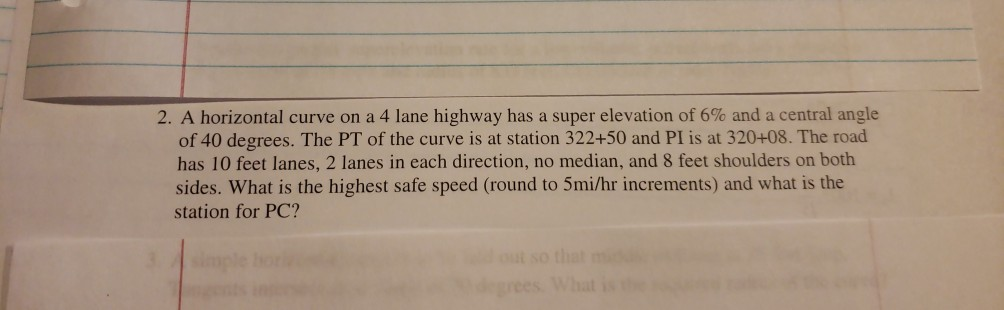2. A horizontal curve on a 4 lane highway has a super elevation of 6% and a central angle of 40 degrees. The PT of the curve is at station 322+50 and PI is at 320+08. The road has 10 feet lanes, 2 lanes in each direction, no median, and 8 feet shoulders on both sides. What is the highest safe speed (round to 5mi/hr increments) and what is the station for PC?

• ### Problem 4. A horizontal curve is being designed through mountainous terrain for a four-lane road with...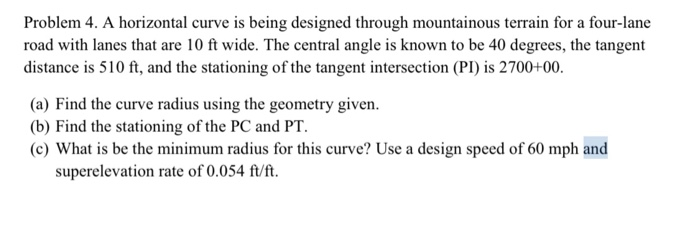Problem 4. A horizontal curve is being designed through mountainous terrain for a four-lane road with lanes that are 10 ft wide. The central angle is known to be 40 degrees, the tangent distance is 510 ft, and the stationing of the tangent intersection (PI) is 2700+00. (a) Find the curve radius using the geometry given. (b) Find the stationing of the PC and PT. (c) What is be the minimum radius for this curve? Use a design speed of...

• ### ON2ohtal curves .The deflecti n angle used when sighting back on the curve during a move up was w...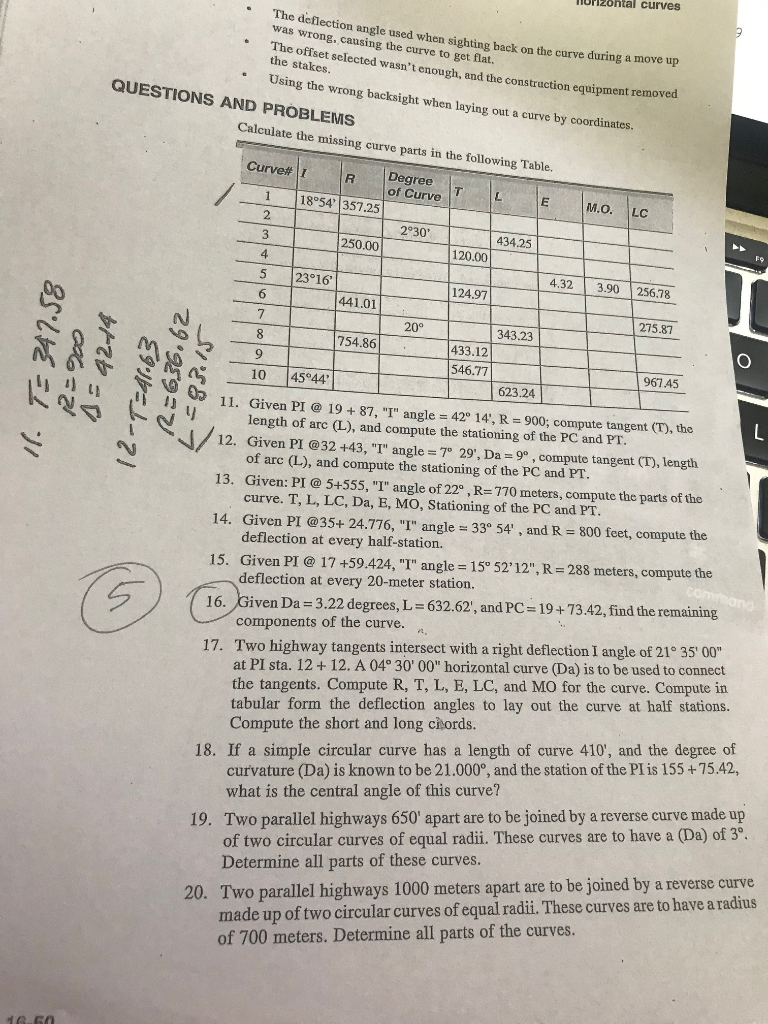Please complete the chart and explain how to solve number 16. Thank you. oN2ohtal curves .The deflecti n angle used when sighting back on the curve during a move up was wrong, causing the curve to get wasn't enough, and the construction equipment removed the stakes. Using the wrong backsight when laying out a curve by QUESTIONS AND PROBLEMS Calculate the missing curve parts in the following Table. Curve# 1 of Curve E M.O. LC 1 18°54' 357.25 2 2930...

• ### Part A. The following information is for a proposed horizontal curve in a new subdivision: PI...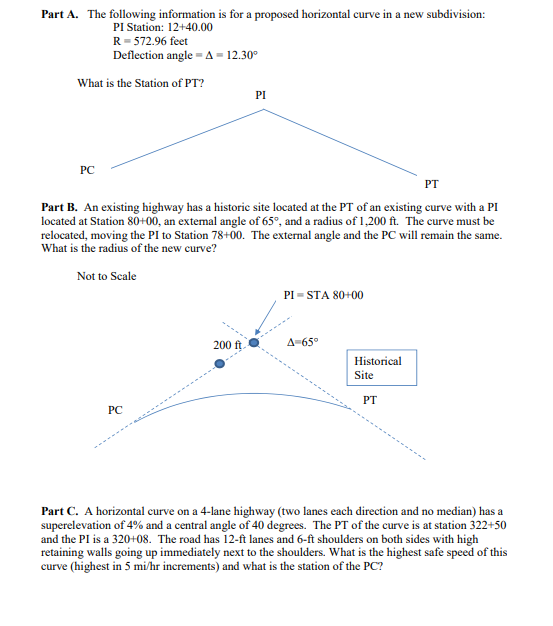Part A. The following information is for a proposed horizontal curve in a new subdivision: PI Station: 12+40.00 R-572.96 feet Deflection angle A-12.30 What is the Station of PT? PI PC PT Part B. An existing highway has a historic site located at the PT of an existing curve with a PI located at Station 80+00, an extemal angle of 65°, and a radius of 1,200 ft. The curve must be relocated, moving the PI to Station 78+00. The external...

• ### Name: Grade: /10 Dec 3, 2018 w ..CA-Horizontal Curve VI FORT WAYNE Tabulate R or D,...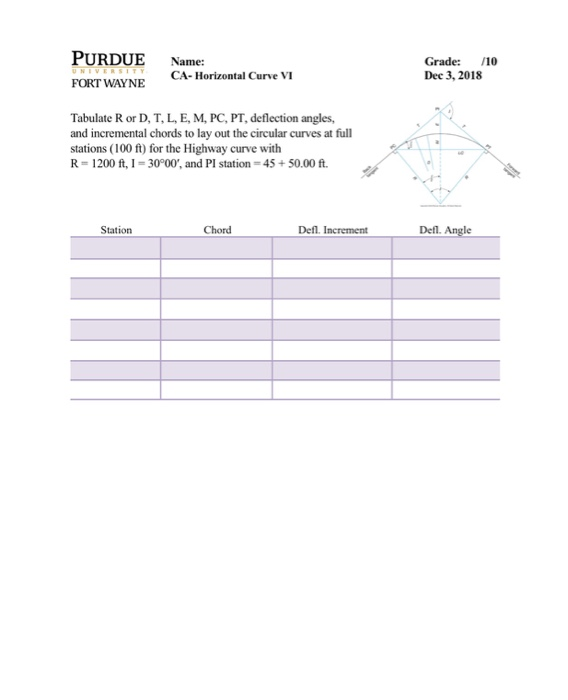Name: Grade: /10 Dec 3, 2018 w ..CA-Horizontal Curve VI FORT WAYNE Tabulate R or D, T, L, E, M, PC, PT, deflection angles, and incremental chords to lay out the circular curves at ful stations (100 ft) for the Highway curve with R 1200 ft, I-30°00, and PI station 45+ 50.00 ft. Station Chord Defl. Increment Defl. Angle

• ### (2 points) A horizontal curve on a two-lane highway (12-ft lanes) is designed for 55 mi/h...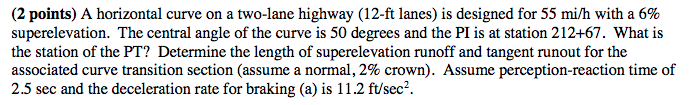(2 points) A horizontal curve on a two-lane highway (12-ft lanes) is designed for 55 mi/h with a 6% superelevation. The central angle of the curve is 50 degrees and the PI is at station 212+67. What is the station of the PT? Determine the length of superelevation runoff and tangent runout for the associated curve transition section (assume a normal, 2% crown). Assume perception-reaction time of 2.5 sec and the deceleration rate for braking (a) is 11.2 ft/sec2

• ### Can you please do this questions? A horizontal curve on a two-lane highway (12 ft lanes)...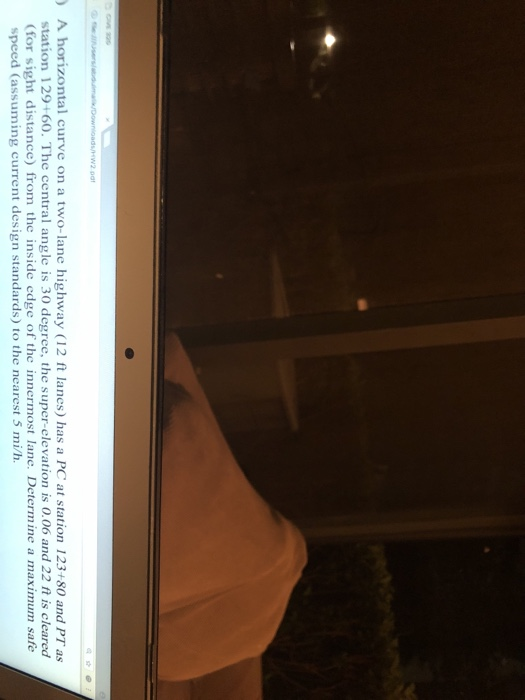Can you please do this questions? A horizontal curve on a two-lane highway (12 ft lanes) has a PC at station 123+80 and PT as station 129+60. The central angle is 30 degree, the super-elevationis 0.06 and 22 ft is cleared (for sight distance) from the inside edge of the innermost lane. Determine a maximum sate speed (assuming current design standards) to the nearest 5 mi/h.